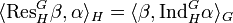Maximum degree of irreducible representation of subgroup is less than or equal to maximum degree of irreducible representation of whole group

(diff) ← Older revision | Latest revision (diff) | Newer revision → (diff)

Statement

Suppose$G$ is a finite group,$H$ is a subgroup, and$K$ is a field whose characteristic does not divide the order of$G$ (we do not require$K$ to be a splitting field, though the splitting field case is of particular interest).

Then, the Maximum degree of irreducible representation (?) of$G$ over$K$ is at least as much as the maximum degree of irreducible representation of$H$ over$K$.

Proof

Proof in characteristic zero

Given:$G$ is a finite group,$H$ is a subgroup, and$K$ is a field of characteristic zero.$m$ is the maximum of the degrees of irreducible representations of$H$ over$K$.

To prove:$G$ has an irreducible representation over$K$ of degree at least$m$.

Proof:

Step no. Assertion/construction Facts used Given data used Previous steps used Explanation
1 Let$\alpha$ be the character of an irreducible representation of$H$ of degree$m$.$m$ is the maximum of degrees of irreducible representations of$H$.
2 Let$\beta$ be the character of an irreducible representation of$G$ arising as a subrepresentation of$\operatorname{Ind}_H^G\alpha$.$H$ is a subgroup of the finite group$G$ Step (1)
3 The inner product$\langle \beta, \operatorname{Ind}_H^G \alpha \rangle_G$ is a positive integer, because$\beta$ is the character of a subrepresentation of the representation affording$\operatorname{Ind}_H^G\alpha$. (Note: Over a splitting field, the inner product is equal to the multiplicity, but in general, it could be bigger if$\beta$ is not absolutely irreducible). Fact (1) To make sense of positive integer, we need$K$ to have characteristic zero. Step (2)
4$\langle \operatorname{Res}^G_H \beta, \alpha \rangle_H = \langle \beta, \operatorname{Ind}_H^G \alpha \rangle_G$. Fact (2) Fact-direct
5$\langle \operatorname{Res}^G_H \beta, \alpha \rangle_H$ is a positive integer, indicating that$\operatorname{Res}^G_H \beta$ contains at least one copy of$\alpha$. (Note again: Over a splitting field, the inner product yields the multiplicity, but in general, it could be bigger if$\alpha$ is not absolutely irreducible). Steps (3), (4)
6 The degree of$\beta$ is at least as much as that of$\alpha$. Step (5) [SHOW MORE]
7 The proof is done:$\beta$ is the desired character and the representation realizing it is the desired representation. Steps (2), (6) Step-combination-direct